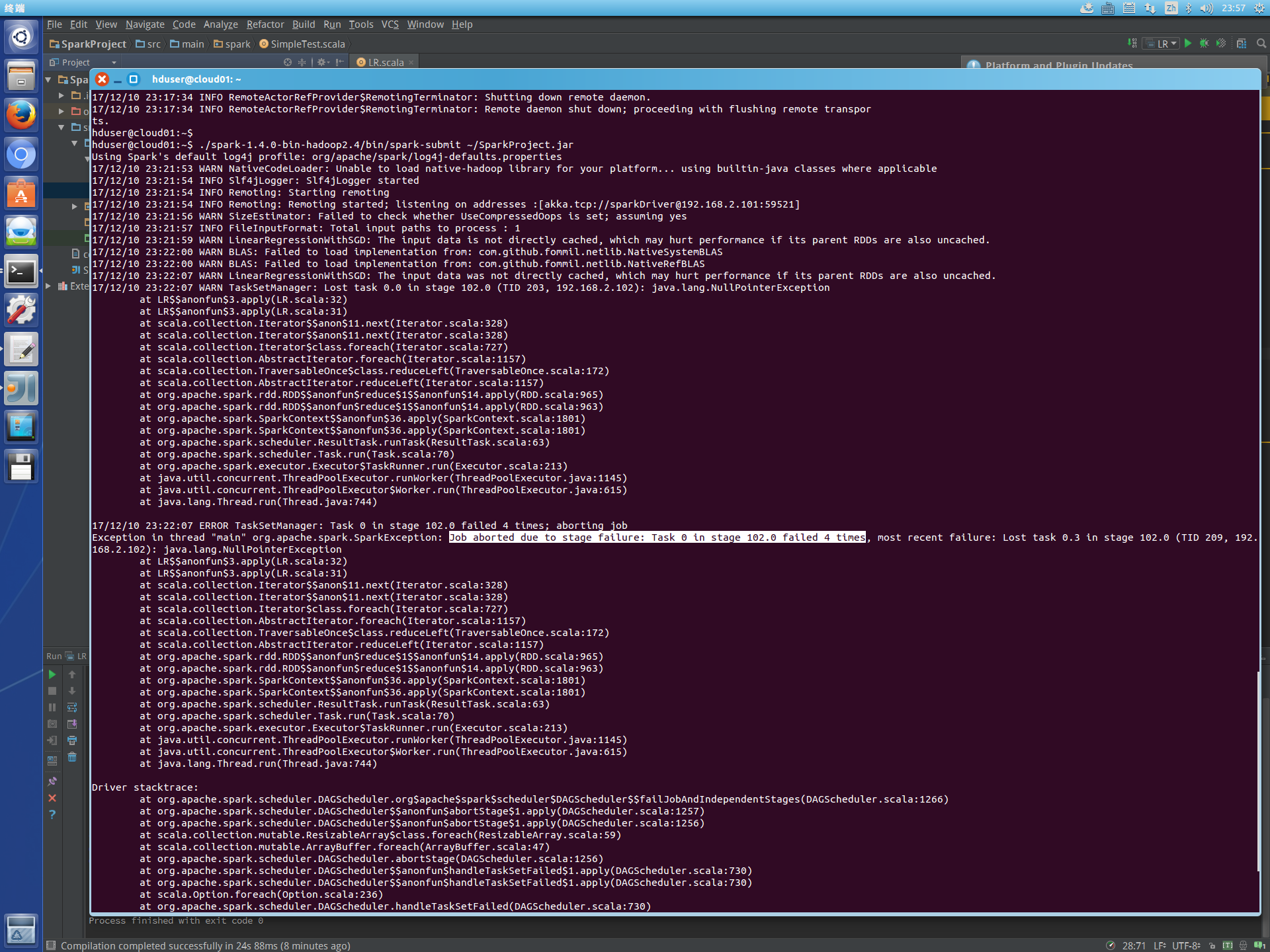Spark部署不知为何出错 80Cpackage spark

import org.apache.spark.{SparkConf,SparkContext}
import org.apache.spark.mllib.regression.LinearRegressionWithSGD
import org.apache.spark.mllib.regression.LabeledPoint
import org.apache.spark.mllib.linalg.{Vectors,Vector }
import org.apache.log4j.{Level, Logger}

object LR extends App{
Logger.getLogger("org.apache.spark").setLevel(Level.WARN)
Logger.getLogger("org.eclipse.jetty.server").setLevel(Level.OFF)

val conf = new SparkConf().setAppName("LinearRegressionTest Yunjisuan")
.setMaster("spark://192.168.2.101:7077")
// .setMaster("local")
val sc = new SparkContext(conf)
// 加载和解析数据文件
val data = sc.textFile("hdfs://cloud01:9000/user/hduser/input/lpsa.data")
val parsedData = data.map { line =>
val parts = line.split(",")
LabeledPoint(parts(0).toDouble, Vectors.dense(parts(1).split(" ").map(x => x.toDouble)))
}
//设置迭代次数并进行训练
val numIterations = 100
val model = LinearRegressionWithSGD.train(parsedData, numIterations)

//统计回归错误的样本比例
val valuesAndPreds = parsedData.map { point =>
val prediction = model.predict(point.features)
(point.label, prediction)

}
val MSE = valuesAndPreds.map{ case(v, p) => math.pow((v - p), 2)}.reduce(_ + _)/valuesAndPreds.count
println("training Mean Squared Error = " + MSE)

val d= Vectors.dense(1.0, 0.0, 0.50,0.32,0.565,0.5,0.7,0.121)
val rdd=sc.makeRDD(Array(Vectors.dense(1.0, 0.0, 0.50,0.32,0.565,0.5,0.7,0.121),Vectors.dense(2.0, 1.0, 1.50,1.32,0.565,4.5,0.7,0.1)))
val prediction = model.predict(d)
val predictions=model.predict(rdd)
println(prediction)
predictions.foreach(println)
}

3个回答hjzdx 能否再具体一些，哪里配置有问题，谢谢！
2 年多之前 回复hjzdx 能否再具体一些，哪里配置有问题，谢谢！
2 年多之前 回复